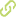# Learning discrete probabilistic models for application in multiple faults detection•
• Overview
•
•
• View All
•

### Abstract

• We present a framework to detect faults in processes or systems based on probabilistic discrete models learned from data. Our work is based on a residual generation scheme, where the prediction of a model for process normal behavior is compared against measured process values. The residuals may indicate the presence of a fault. The model consists of a general statistical inference engine operating on discrete spaces, and represents the maximum entropy joint probability mass function (pmf) consistent with arbitrary lower order probabilities. The joint pmf is a rich model that, once learned, allows us to address inference tasks, which can be used for prediction applications. In our case the model allows the one step-ahead prediction of process variable, given its past values. The relevant dependencies between the forecast variable and past values are learnt by applying an algorithm to discover discrete bayesian network structures from data. The parameters of the statistical engine are also learn by an approximate method proposed by Yan and Miller. We show the performance of the prediction models and their application in power systems fault detection.

### Publication date

• December 1, 2008